## Analogy

#### Analogy

Direction: Select the related figure from the given alternatives.

1. Find the question mark ? figure from answer figure.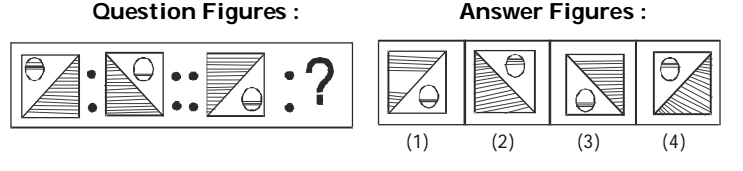1. The second figure is the mirror image of the first figure.

##### Correct Option: C

The second figure is the mirror image of the first figure.

1. Find the question mark ? figure from answer figure.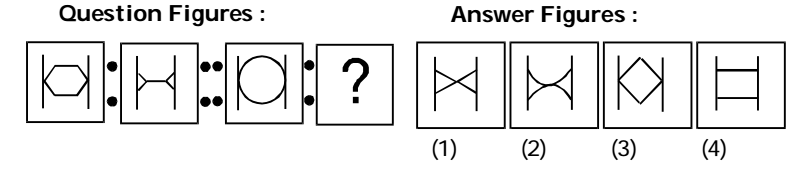1. From first figure to second figure the right and the left parts of design get separated and then inverted. Similarly, from the third figure to the answer figure the circle will be divided into two equal halves and then they will be inverted.

##### Correct Option: B

From first figure to second figure the right and the left parts of design get separated and then inverted. Similarly, from the third figure to the answer figure the circle will be divided into two equal halves and then they will be inverted.

1. Find the question mark ? figure from answer figure.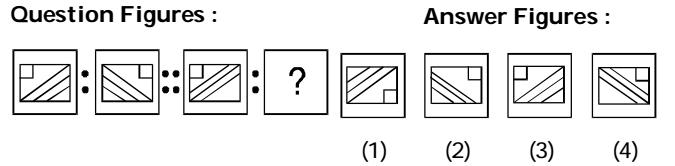1. The second figure is the mirror image of the first figure.

##### Correct Option: D

The second figure is the mirror image of the first figure.

1. Find the question mark ? figure from answer figure.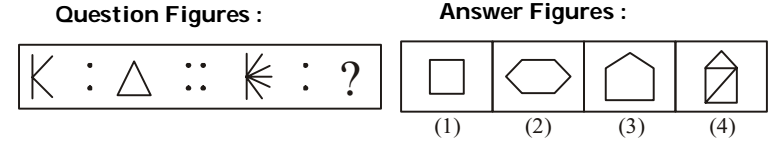1. There are three line segments in the first figure and these three line segments are converted into a three-sided geometrical figure, i.e., a triangle. Similarly, six line segments would be converted into a hexagon.

##### Correct Option: B

There are three line segments in the first figure and these three line segments are converted into a three-sided geometrical figure, i.e., a triangle. Similarly, six line segments would be converted into a hexagon.

1. Find the question mark ? figure from answer figure.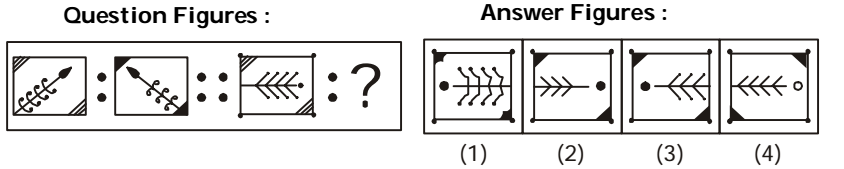1. From first figure to second figure one curve is deleted, the two corners of square become shaded and the main design shifts its side.

##### Correct Option: C

From first figure to second figure one curve is deleted, the two corners of square become shaded and the main design shifts its side.